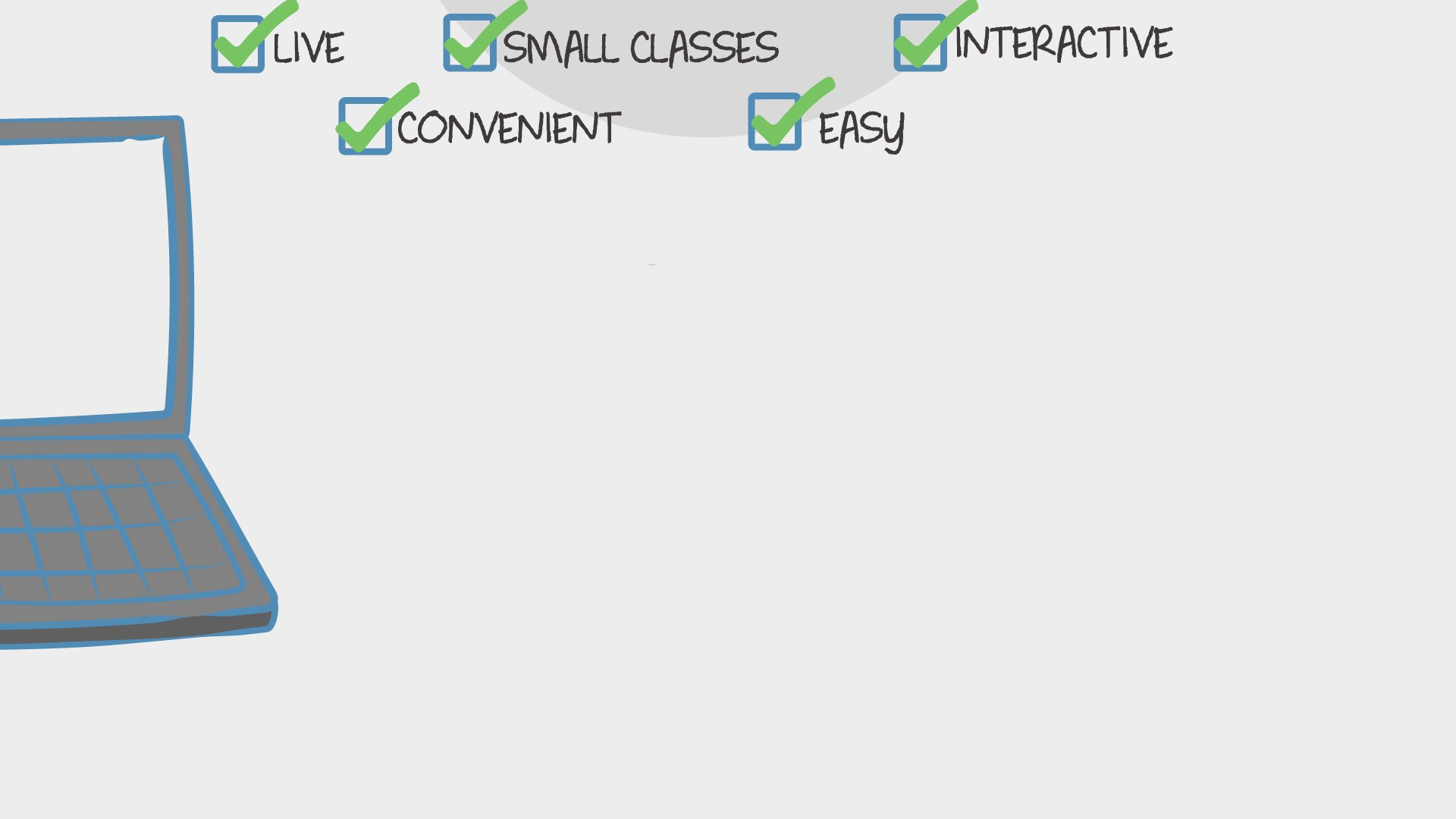Find Classes
Teach

Math

# Algebra 1, Semester 2, With a Licensed Math Teacher

In this fifteen week course we will cover the second half of Common Core Algebra 1.Mrs. Mary
15 total reviews for this teacher
New class
There are no upcoming classes.45 minutes
per class
4x per week
over 15 weeks
10-15
year olds
8-15
learners per class
per learner - per class

### How does a "Multi-Day" course work?

Meets multiple times at scheduled times
Live video chats, recorded and monitored for safety and quality
Discussions via classroom forum and private messages with the teacher
Great for engaging projects and interacting with diverse classmates from other states and countries### How Outschool Works### There are no open spots for this class.

You can request another time or scroll down to find more classes like this.

## Description

### Class Experience

`This class is taught in English.`
```The students will:
- Find the domain and range of functions from graphs or tables.
- Determine whether relations are functions.
- Graph discrete and continuous data.
- Determine whether functions have a discrete or continuous domain.
- Write linear functions from graphs or tables.
- Solve linear functions.
- Write and solve systems of linear equations by graphing, substitution and elimination.
- Solve systems of linear equations having no solution or infinitely many solutions.
- Solve linear equations by graphing a system of linear equations.
- Write and graph systems of linear inequalities in two variables.
- Simplify and evaluate square roots.
- Simplify radical expressions.
- Simplify expressions using the properties of exponents.
- Simplify expressions with rational exponents.
- Identify, evaluate, and graph exponential functions.
- Solve exponential equations algebraically and graphically.
- Write, interpret, and graph exponential functions.
- Identify, write and graph exponential growth and decay functions.
- Write equations for geometric and arithmetic sequences.
- Write the terms of recursively defined sequences.
- Write recursive equations for sequences.
- Find the degrees of monomials.
- Classify, add, subtract and multiply polynomials.
- Use patterns to multiply polynomials.
- Solve polynomial equations in factored form.
-  Factor polynomials using the greatest common factor.
- Solve polynomial equations by factoring.
- Factor trinomials in all forms
- Factor special products
- Factor polynomials by grouping.
- Identify characteristics of quadratic functions.
- Graph quadratic functions.
- Find the foci of parabolas.
- Write equations of parabolas with vertices at the origin given the foci.
- Graph quadratic functions
- Find the axes of symmetry and the vertices of
graphs.
- Graph quadratic functions in vertex form.
- Find maximum and minimum values.
- Identify linear, quadratic, and exponential functions using graphs or tables.
- Compare graphs of linear, quadratic, and exponential functions.
- Solve quadratic equations by graphing, taking the square roots, completing the square, and using the quadratic formula.
- Use discriminants to determine the number of real solutions of quadratic equations.
- Solve systems of linear and quadratic equations.
-  Graph and compare square root functions.
- Simplify radical expressions.
- Solve square root equations, including those with square roots on both sides.
- Identify extraneous solutions.
- Use the Pythagorean Theorem to find missing side lengths of right triangles.
- Identify right triangles.
- Find distances between two points.
-  Identify, write and graph direct and inverse variation equations and rational functions.
- Identify asymptotes.
- Simplify rational expressions.
- Add, subtract, multiply and divide rational expressions.
- Divide polynomials by monomials and binomials.
- Solve rational equations.
- Calculate measures of central tendency and dispersion.
- Display and analyze data.```
`I am a licensed mathematics teacher and have thirteen years of classroom experience teaching Pre-Algebra, Algebra and Geometry. My students have consistently experienced exceptional growth in comparison to local, state and national metrics.`
`Homework with an answer key will be provided with each lesson; it is optional be strongly recommended. I will be available for homework help sessions daily by request.`
```The students should be ready for each class with:
Formatted notes printed (provided)
Pencil and eraser
calculator
Whiteboard with a coordinate plane for extra practice when time allows```
`Students will be formatively assessed during each lesson with observation and targeted questioning. Summative assessments and grades will be available upon request.`
`3 hours per week in class, and an estimated 2 - 4 hours per week outside of class.`
`Students should have successfully completed the first semester of Algebra 1.`

## Teacher

`Hello! Let me introduce myself. I am a life long learner that found my vocation in education. I love working with children because they share my curiosity about everything. I am a licensed elementary/middle school teacher and a licensed math... `